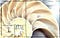# Erdős’s Indestructible Graph

## Math Snack #4

Welcome to Math Snacks, a series of interesting and profound mathematical results in bite-sized form!

This snack explores several interesting properties of an infinite graph.

Definitions:

A graph G is a pair (V, E) of vertices and edges. A Rado Graph (or Erdős–Rényi Graph) is a random infinite graph constructed as follows:

Let V be a countably infinite set of vertices {v1, v2, …, vn, …}. For each pair of vertices, flip a (fair or unfair) coin. Connect the vertices with an edge if and only if the coin lands on heads.

Graphs G1 and G2 are said to be…

# Cantor’s Realization

## Math Snack #3

Welcome to Math Snacks, a series of interesting and profound mathematical results in bite-sized form!

This snack reveals that not all infinities are the same.

Definitions:

A power set of a set S (denoted ℘(S)) is the set of all possible distinct subsets of S (sets that can be formed from the elements of S). For instance, consider the set S = {0, 1, 2}. Then ℘(S) = {{}, {0}, {1}, {2}, {0, 1}, {0, 2}, {1, 2}, {0, 1, 2}}.

The cardinality of a set is the number of elements in that set. The cardinality of S is denoted…

# The Fibonacci Formula

## Math Snack #2

Welcome to Math Snacks, a series of interesting and profound mathematical results in bite-sized form!

This snack concerns the Fibonacci Sequence and a surprising way to define it.The Fibonacci Sequence is found frequently in nature…shells, flowers, galaxies, hurricanes…

Definitions:

A function is real-valued and continuous if, roughly speaking, its graph is a single unbroken curve whose domain is the entire real number line.

A recurrence relation is an equation that recursively defines a sequence of values. A simple example is the following: the first term of a recurrence relation F is 0, and the v-th term of F is the (v-1)-th term plus 1. …

# Pólya’s Random Walks

## Math Snack #1

Welcome to Math Snacks, a series of interesting and profound mathematical results in bite-sized form!

This snack concerns random walks on n-dimensional lattices.

Definitions:

For our purposes, a lattice is a regular arrangement of points in Euclidean space. In this snack, a 1D integer lattice can be thought of as the integer line … -2, -1, 0, 1, 2, …, a 2D integer lattice can be thought of as the integer grid of all integer tuples (i, j), …, an n-dimensional integer lattice can be thought of as all integer tuples of dimension n.

Also for our purposes, a random…

# Message in a Message

## Exploring Deep Steganography for Language

Ste·ga·no·graph·y / stegəˈnägrəfi / (noun): the practice of concealing messages or information within other nonsecret text or data.

Synopsis: it’s possible to effectively conceal sensitive information inside language without raising eavesdropper suspicion using deep learning. This has important implications for secure communication.

Edit 09/06/20: Check out the end of the article for a machine-generated summary! More to come in that direction soon. :)

Steganography has been used for ages to communicate information in a hidden manner. A natural question: how is this different from cryptography? Isn’t cryptography a very well-studied field in which two individuals aim to share information with…## Josh Payne

Co-Founder of AccessBell, MSCS/MBA at Stanford; past: Ciphense, Marlin Protocol, IBM Research, Apfyt. Musician, composer, researcher, pennyboarder, builder